Browse by Topic
Related Topics

# Mathematica Q&A: Plotting Trig Functions in Degrees

Got a question about Mathematica? The Wolfram Blog has answers! We’ll regularly answer selected questions from users around the web. You can submit your question directly to the Q&A Team using this form.

This week’s question comes from Brian, who is a part-time math teacher:

How do you plot trigonometric functions in degrees instead of radians?

Trigonometric functions in Mathematica such as Sin[x] and Cos[x] take x to be given in radians: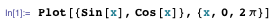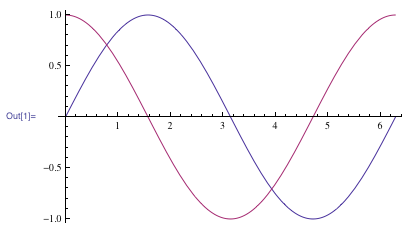To convert from degrees to radians, multiply by π ⁄ 180. This special constant is called Degree in Mathematica.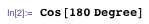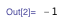The symbol ° is a handy shorthand for Degree and is entered as Esc-d-e-g-Esc. You can also find this symbol in the Basic Math Assistant palette in the Palettes menu of Mathematica.

Using either Degree or °, you can plot trigonometric functions in degrees: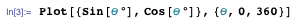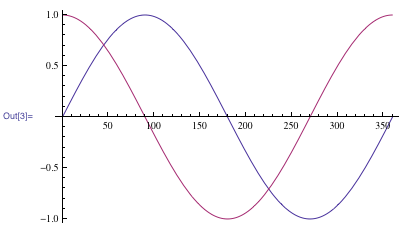That answers the main question, but here’s a related hint.

When plotting trigonometric functions in degrees, you might also want to manually specify exactly where Mathematica draws tick marks. You can do this using the Ticks option: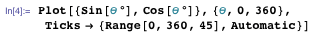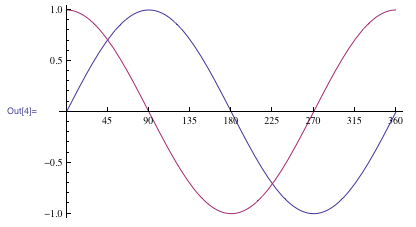(Here, Range[0, 360, 45] specifies the tick marks on the x axis, and Automatic uses the default tick marks on the y axis.)

The Ticks option is very flexible. You can specify where tick marks are drawn, what labels they should have, how long they are, and even colors and styles.

Download the Computable Document Format (CDF) file for this post to see how to get the custom tick marks used in this plot: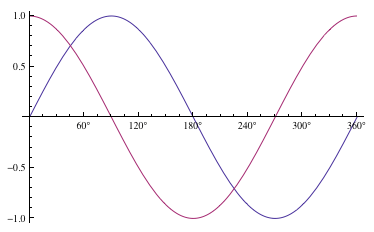If you have a question you’d like to see answered in this blog, you can submit it to the Q&A Team using this form.

Download the CDF file